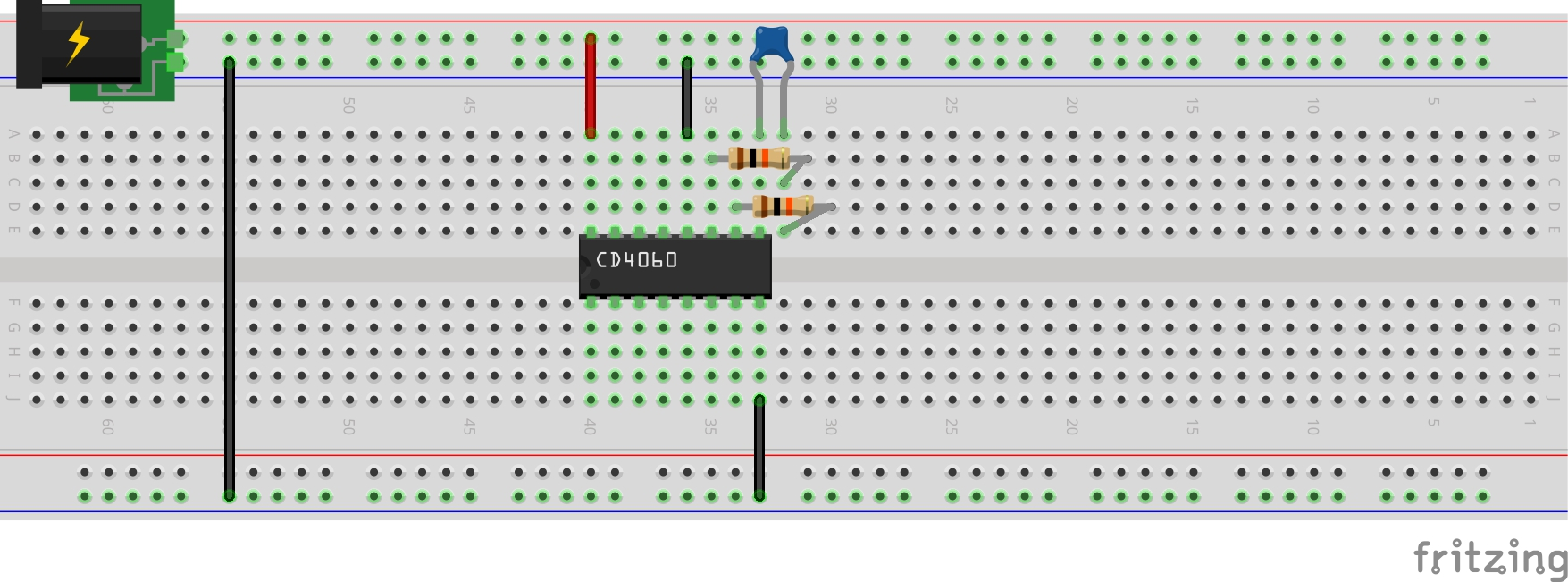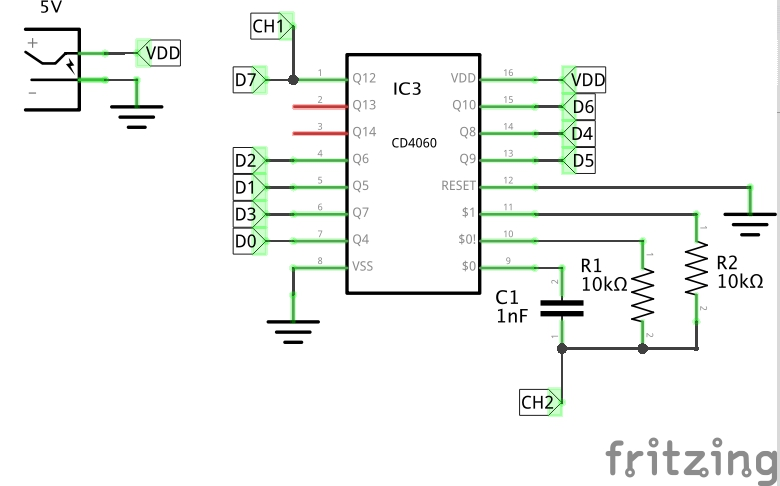# #293 CD4060/RCOscillator

Exploring the behaviour of the CD4060 ripple counter driven by an RC oscillator.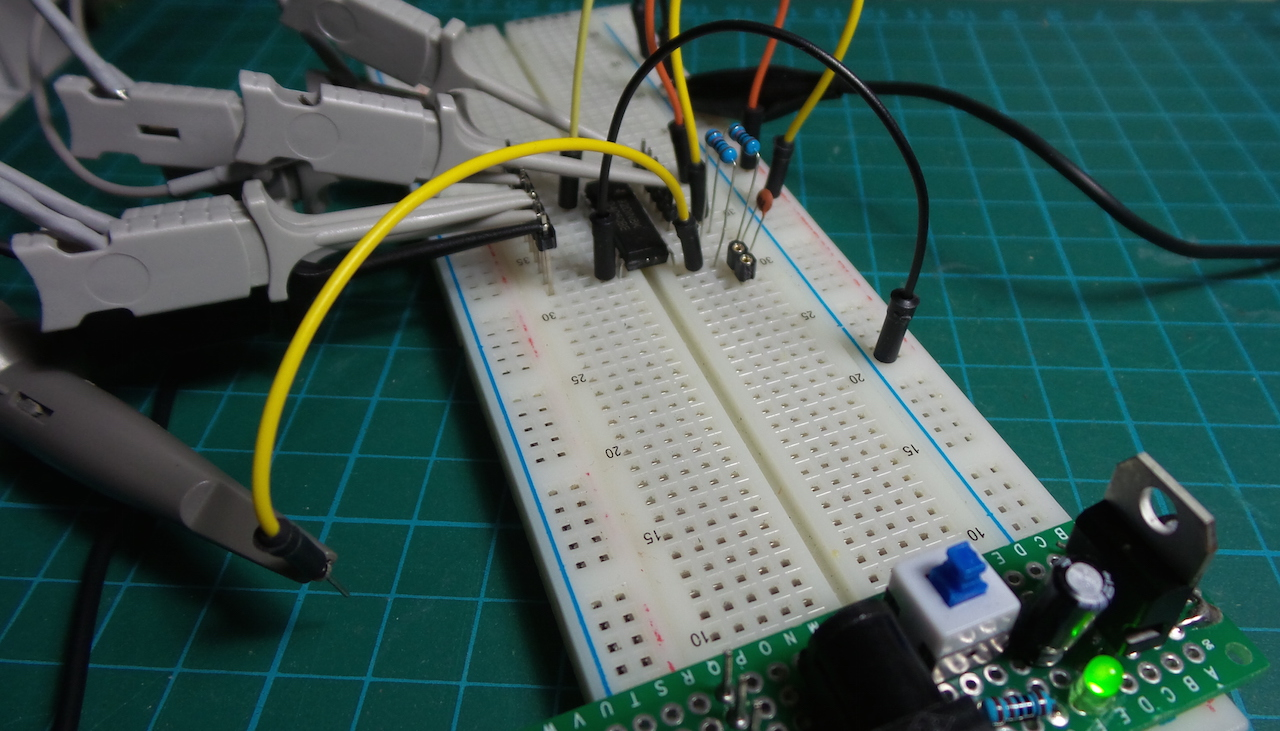The CD4060 datasheet describes an RC oscillator configuration for self-driving the ripple counter. A 50% duty cycle is achieved when R1 = R2.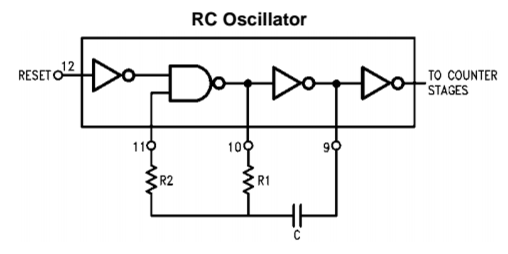In this test I’m using R1 = R2 = 10kΩ, and C1 = 1nF. During charge/discharge, the equivalent resistance is 10kΩ|10kΩ i.e. 5kΩ, so the time constant for the rising and falling phases is 5µs (200kHz).

Thus for a near complete charge/discharge of 5 time constants, we’d expect an oscillation of around 40kHz. In practice, I’m measuring 38.7kHz .. so the approximation appears to be pretty close.

Here’s a trace of the oscillator (tapped at the net marked CH2 in the schematic):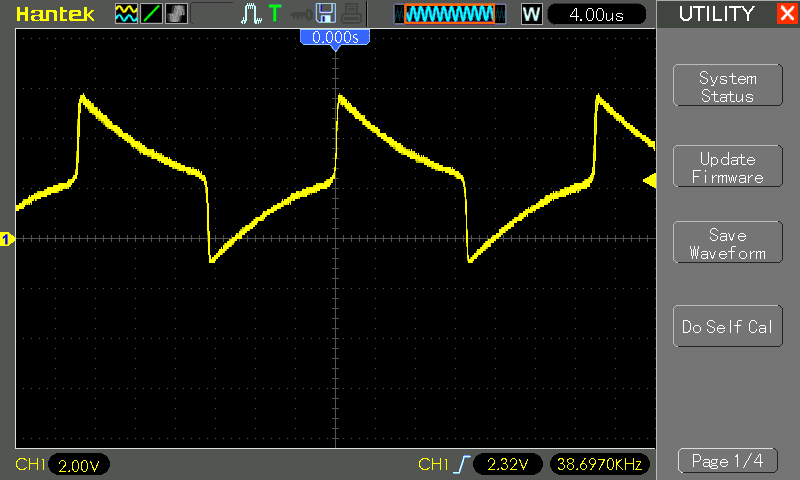The reset pin 12 should be pulled low to ensure stable operation. If left floating it can cause spurious results such as picking up 50/60Hz oscillation.

### Ripple Counter

The CD4060 is a 14 stage ripple counter constructed of RS flip-flop units - see the functional diagram from the datasheet: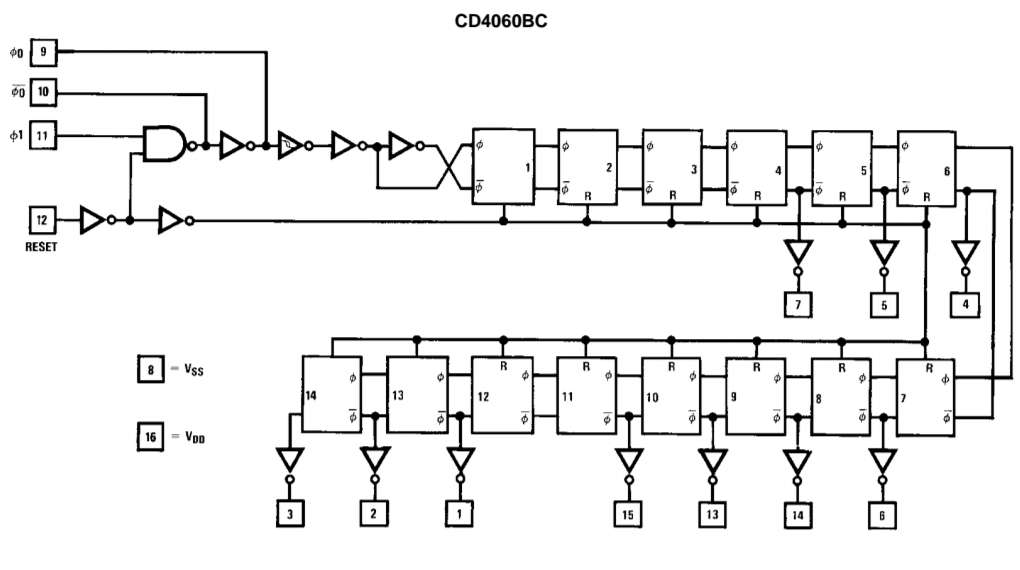The input signal passes 4 stages before the first output is tapped (Q4). Thus the first (Q4) output signal divides the input frequency by a factor of 2^4

Stage 11 (Q11) of the ripple counter is also not exposed on a pin.

The lack of Q0-3 and Q11 is I think just pin economics so it all fits it in a DIP16 package.

The performance is summarised in the table and scope capture below.

Signal Scope Frequency (theory) Frequency (actual) Note
CH1 CH1 9.765Hz 10Hz = D7
CH2 CH2 40kHz 38.7kHz f-input
Q4 D0 2.5kHz 2.5kHz f-input/2^4
Q5 D1 1.25kHz 1.25KHz f-input/2^5
Q6 D2 625Hz 623Hz f-input/2^6
Q7 D3 312.5Hz 312Hz f-input/2^7
Q8 D4 156.25Hz 155Hz f-input/2^8
Q9 D5 78.125Hz 78Hz f-input/2^9
Q10 D6 39.06Hz 39Hz f-input/2^10
Q12 D7 9.765Hz 10Hz f-input/2^12
Q13   4.883Hz 5Hz f-input/2^13
Q14   2.441Hz 2Hz f-input/2^14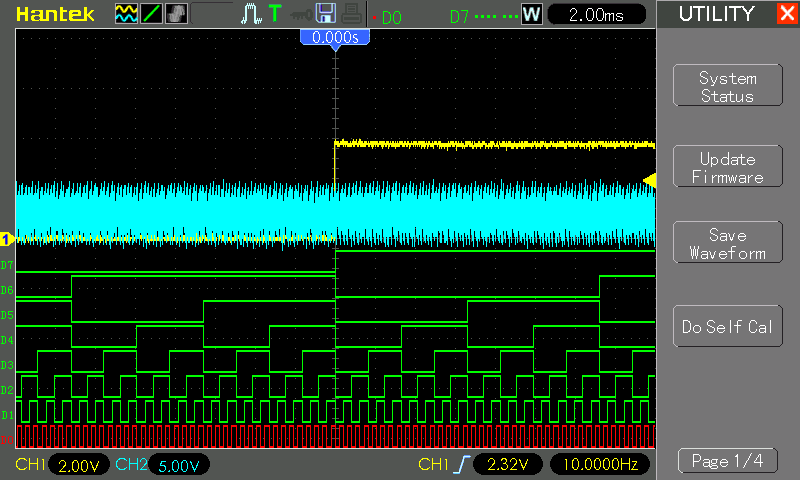## Construction